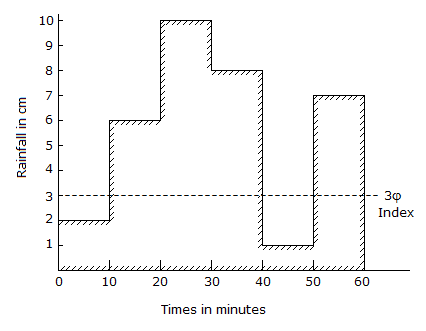# Civil Engineering - Water Resources Engineering - Discussion

### Discussion :: Water Resources Engineering - Section 2 (Q.No.29)

29.

From the data of the rain storm shown in the below figure, the value of Windex is[A]. 1.5 cm/hour [B]. 2 cm/hour [C]. 2.5 cm/hour [D]. 2 cm/hour.

Explanation:

No answer description available for this question.

 Mukesh Maurya said: (Jul 22, 2016) W - index = total average precipitation - phi ndex.

 Charu said: (Aug 10, 2016) Please explain it.

 Er Shailza Kant Sagar said: (Oct 7, 2016) The formula is is P - Q/T for w index.

 Omar said: (Jun 7, 2017) Total average precipitation = ( 2 +6+10+8+1+7)/6 = 5.6 ~ 5.5 -3 = 2.5 cm/hour.

 Chhaya said: (Sep 28, 2017) (2+3+3+3+1+3)/6=2.5.

 D.Paul said: (Dec 18, 2017) Total average precipitation = (2 +6+10+8+1+7)/6 = 5.6 ~ 5.5 -3 = 2.5 cm/hour.

 Raaj said: (Aug 12, 2018) Thank you all for giving the explanation.

 Deepak Baviskar said: (Dec 20, 2019) Windex = Total Precipitation - Runoff. = 5.5 - 3. = 2.5 cm/hr.